Main Content

# GPU Code Generation for Lane Detection in Simulink

This example shows how to generate CUDA® code for a Simulink® model that can detect and output lane marker boundaries on an image. This example takes RGB image as an input and uses the `imresize` (Image Processing Toolbox), `rgb2gray`, `ordfilt2` (Image Processing Toolbox), `hough` (Image Processing Toolbox), `houghpeaks` (Image Processing Toolbox), and `houghlines` (Image Processing Toolbox) functions that are part of Image Processing Toolbox™ to detect lane markings. This example closely follows Lane Detection on the GPU by Using the houghlines Function.

This example illustrates the following concepts:

• Model a lane detection application in Simulink by using image processing functions.

• Configure the model for GPU code generation.

• Generate a CUDA executable for the Simulink model.

### Third-Party Prerequisites

Required

This example generates CUDA MEX and has the following third-party requirements.

• CUDA enabled NVIDIA® GPU and compatible driver.

Optional

For non-MEX builds such as static, dynamic libraries or executables, this example has the following additional requirements.

### Verify GPU Environment

To verify that the compilers and libraries necessary for running this example are set up correctly, use the `coder.checkGpuInstall` function.

```envCfg = coder.gpuEnvConfig('host'); envCfg.BasicCodegen = 1; envCfg.Quiet = 1; coder.checkGpuInstall(envCfg); ```

### Lane Detection using `houghlines` Simulink Model

The Simulink model for lane detection is shown.

```open_system('lane_detection'); ```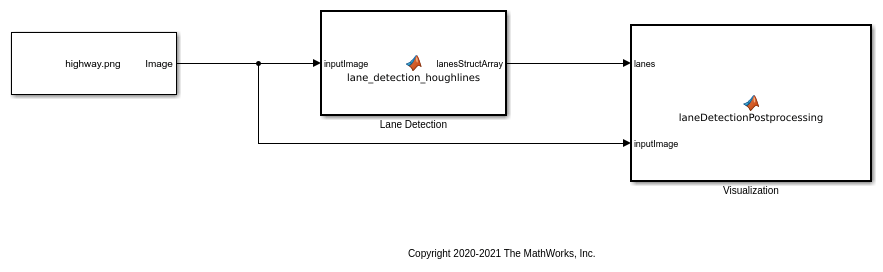The `Lane Detection` subsystem contains a `MATLAB Function` block that takes an intensity image as input and provides detected lanes as output. This function is based on the lane detection algorithm implementation using `houghlines` as described in Lane Detection on the GPU by Using the houghlines Function example. When the model runs, the `Visualization` block displays the lane detected output image.

### Run the Simulation

Open Configuration Parameters dialog box.

In Simulation Target pane, select GPU acceleration.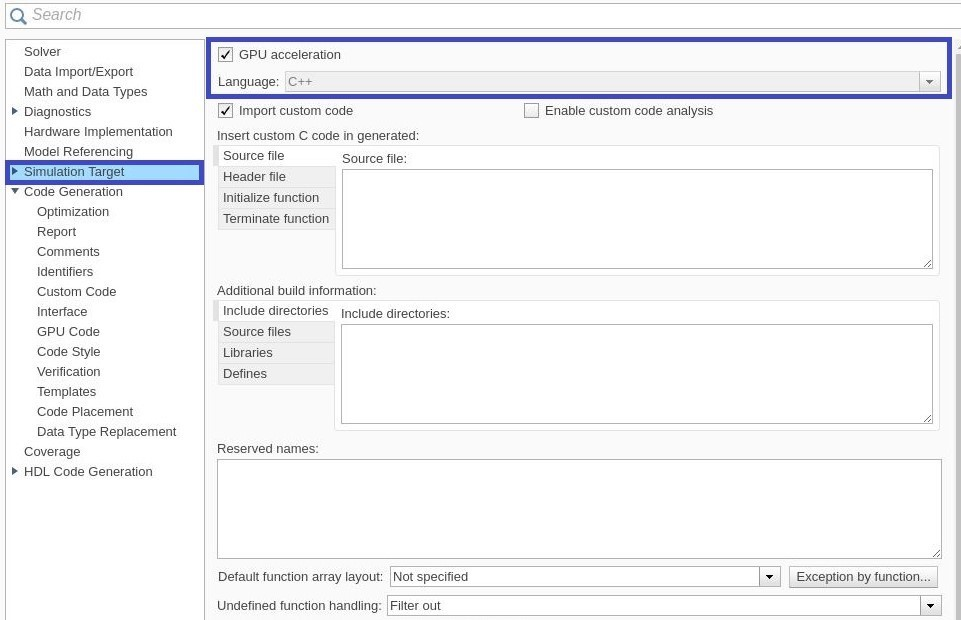Run the simulation in Normal mode.

```set_param('lane_detection', 'SimulationMode', 'Normal'); sim('lane_detection'); ```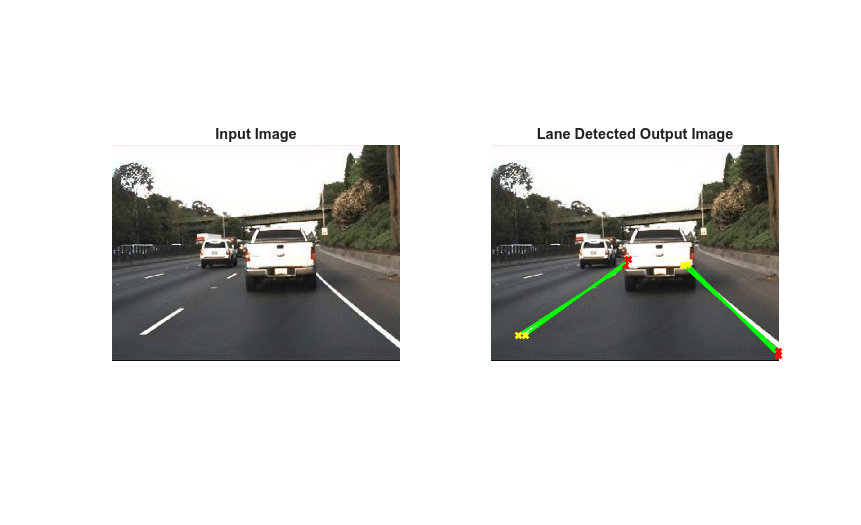### Generate and Build the Simulink Model

In Code Generation pane, select the Language as C++ and enable Generate GPU code.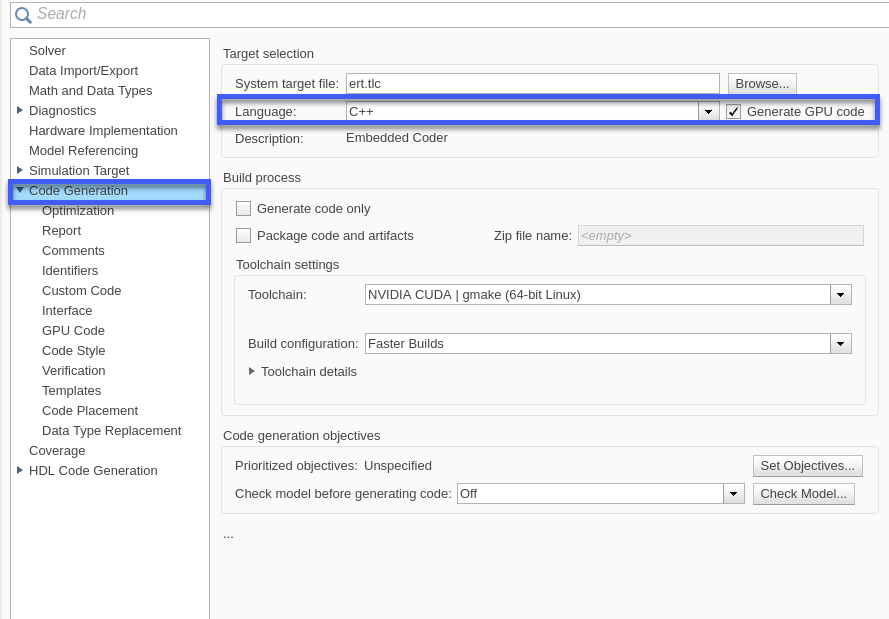Open Simulation Target pane. In the Advanced parameters, enable Dynamic memory allocation threshold in MATLAB functions. For more information, see Dynamic memory allocation in MATLAB functions (Simulink)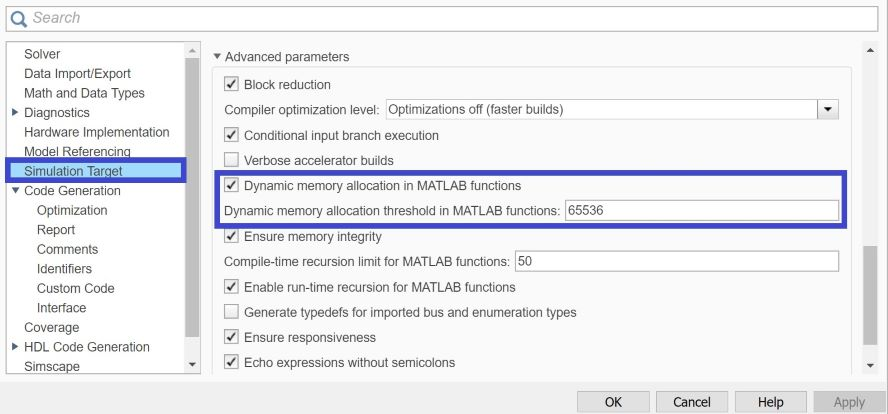Open Code Generation > GPU Code pane. In the subcategory Libraries, enable cuBLAS, cuSOLVER and cuFFT.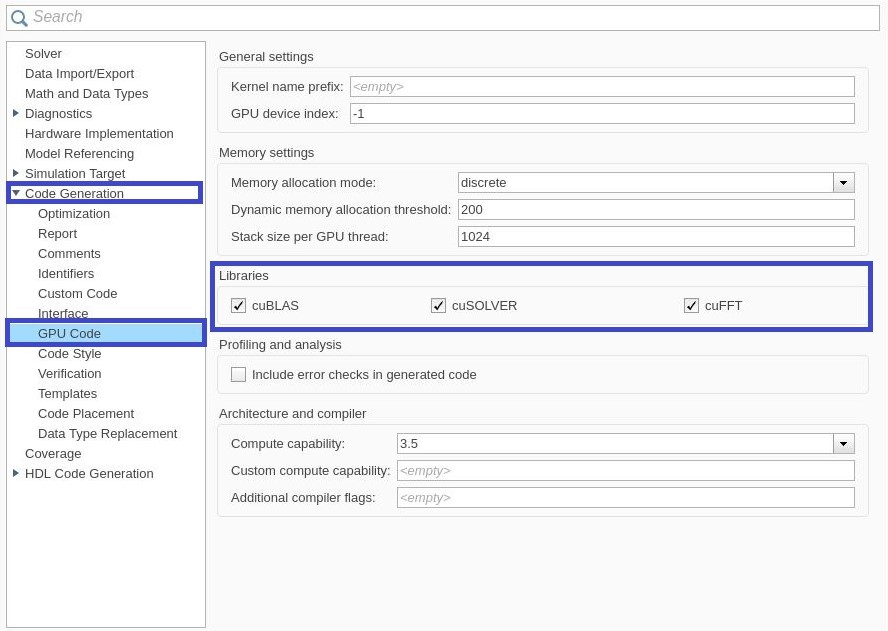Generate and build the Simulink model on the host GPU by using the `slbuild` command. The code generator places the files in a build folder, a subfolder named `lane_detection_ert_rtw` under your current working folder.

```status = evalc("slbuild('lane_detection')"); ```

### Cleanup

Close the Simulink model.

```close_system('lane_detection'); ```

## Support

#### Generating CUDA Code from MATLAB: Accelerating Embedded Vision and Deep Learning Algorithms on GPUs

Download white paper